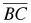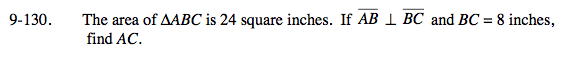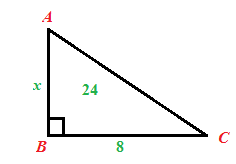Home > A2C > Chapter 9 > Lesson 9.3.1 > Problem9-130

9-130.

The area of ΔABC is 24 square inches. Ifand BC = 8 inches, find AC. Homework Help ✎Begin by drawing and labeling a diagram.Use an area equation.

$\frac{8\textit{x}}{2}=24$

x = 6 inches, what is the length of AC?